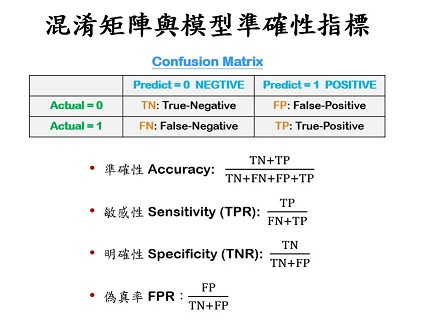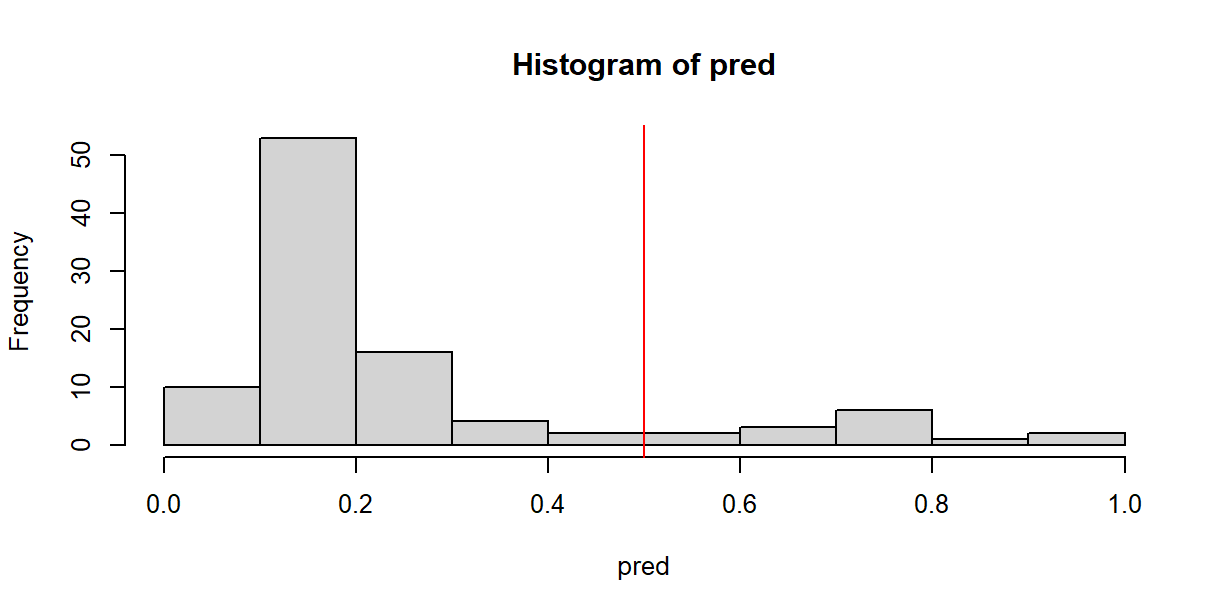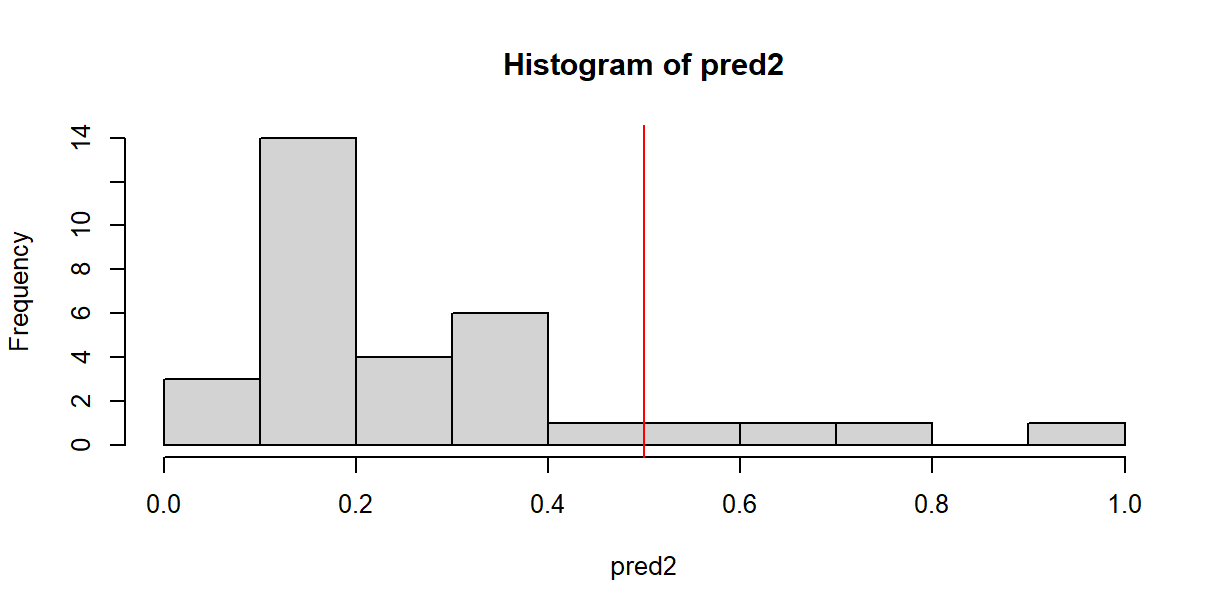CASE : Quality of Medical Care

``````pacman::p_load(caTools, ggplot2, dplyr)
set.seed(88)
split = sample.split(D\$PoorCare, SplitRatio = 0.75)  # split vector
TR = subset(D, split == TRUE)
TS = subset(D, split == FALSE)
glm1 = glm(PoorCare ~ OfficeVisits + Narcotics, TR, family=binomial)
summary(glm1)``````

### 【A】Accuracy with Fix Threshold (`p = 0.5`)Fig 10C.1 - Confusion Matrix & Model Accuracy

##### Training Data

Predicted Probability (Training)

``````par(cex=0.8)
pred = predict(glm1, type="response")
hist(pred)
abline(v=0.5, col='red')``````Confusion Matrix (Training)

``````cmx = table(
Acture=TR\$PoorCare,   # actual y
Predict=pred > 0.5    # predicted y with threshold p=0.5
)
cmx``````
``````      Predict
Acture FALSE TRUE
0    70    4
1    15   10``````

Accuracy Metrics (Training)

``````# define a helper function for repeated usages
AccuracyMetrices = function(x, k=3) { c(
accuracy = sum(diag(x))/sum(x),                    #
sensitivity = as.numeric(x[2,2]/rowSums(x)),    #
specificity = as.numeric(x[1,1]/rowSums(x))     #
) %>% round(k) }
# it takes a confusion matrix and product a vector of accuracy measures
AccuracyMetrices(cmx)``````
``````   accuracy sensitivity specificity
0.808       0.400       0.946 ``````

##### Testing Data

Predicted Probability (Testing)

``````par(cex=0.8)
pred2 = predict(glm1, newdata=TS, type="response")
hist(pred2, 10)
abline(v=0.5, col='red')``````Confusion Matrix (Testing)

``````cmx2 = table(Acture=TS\$PoorCare, Predict=pred2 > 0.5)
cmx2``````
``````      Predict
Acture FALSE TRUE
0    23    1
1     5    3``````

Accuracy Matrices (Testing)

``````# with the helper function, we can compare the training and testing accuracy at once
sapply(list(Train=cmx, Test=cmx2), AccuracyMetrices)``````
``````            Train  Test
accuracy    0.808 0.812
sensitivity 0.400 0.375
specificity 0.946 0.958``````